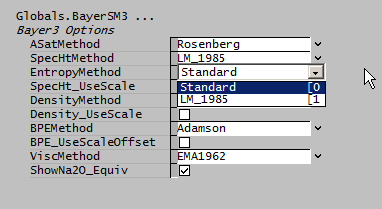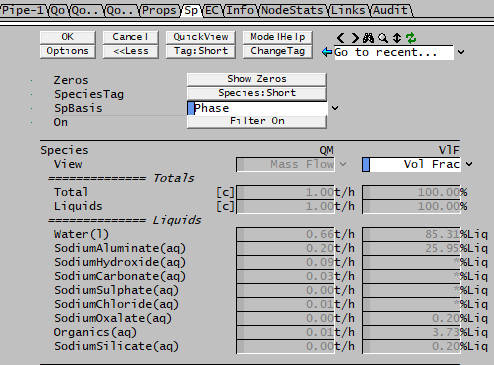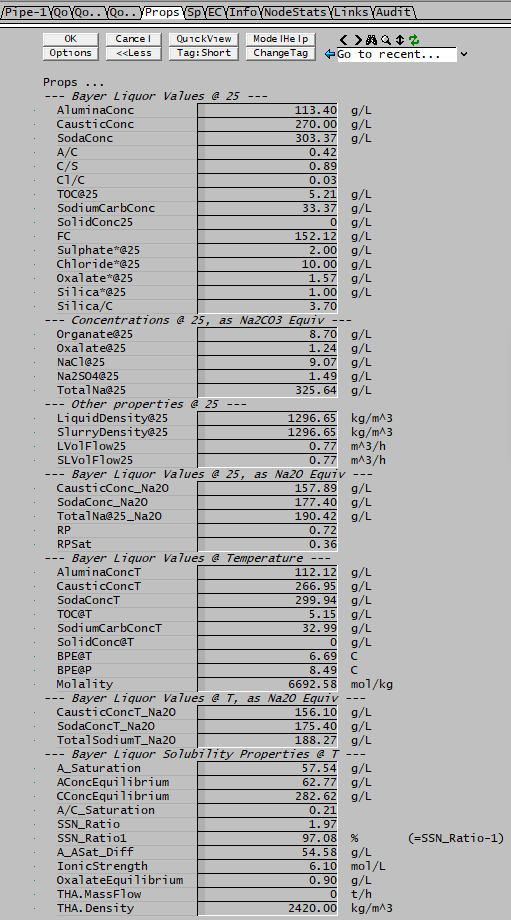# General Bayer Data

Navigation: Main Page -> Models -> Alumina Models

Alumina 3 Bayer Species Model Species Model Theory General Discussion

## General Description

All of the Bayer Properties Species Models implemented in SysCAD are used to calculate the properties of liquors within an Alumina project.

The models use equations defined in the public domain. The model equations can be selected in the Global Method. For information on the equations used, please see Model Theory.

The Bayer property models also generally include

The public domain bayer property models included with SysCAD are:

NOTE: It is recommended that the Alumina3 Bayer Species model and corresponding Precipitation3 model is used rather than the Alumina1 version.

Because of the different Bayer stream species representations it is generally not straight forward to simply convert an Alumina1 (Bayer) to an Alumina3 (Bayer3) model. For information on how to convert the species model, please see Converting Alumina 1 to Alumina3.

All of the properties that are not explicitly calculated by the Bayer species models are calculated using the Standard species model, which uses the mass weighted mean of the liquor (see Standard Species Model).

Refinery specific equations for various thermodynamic properties are often used to create client specific Alumina add-ons.

## Implications of using a Bayer Properties model

### Mixing Streams with different Properties Models

When multiple streams are combined in a unit, and some streams use the Standard Species Model and others use a Bayer Species model, then SysCAD selects the higher level Bayer Properties model for the combined mixture.

### Enthalpy

Hf@0 values will be the same as if using the Standard Species Model. For species other than water and steam, this means that the Hf@25 values entered in the species database will be changed to Hf@0 values using the heat capacities (Cp) values entered in the species database (rather than the bayer liquor heat capacities).
Due to the liquid heat capacity (Cp) being different for a bayer stream, the liquid Hs, Hz and Hf@T values will be different (remember that Hf@T = Hf@0 + Hs and Hs = f(Cp)).

### Entropy

For Builds up to 136, entropy values are not calculated by the Bayer model and hence will be the same as calculated by the Standard Species Model.
In Build 137 we have implemented optional calculation of entropy based on the LM1985 specific heat.$S(T) = S^{25} + \int_{298.15}^T Cp/T dT$

### Caution

• The $S^{25}$ value is the standard species model evaluation of entropy, based on $S^{25}$ values for the individual species in Bayer liquor. There is little or no data for these, in particular for the pseudo-organic species and others; these usually just default to zero.
• Without accurate reference data, the absolute value of entropy as calculated is suspect and should be used with caution.
• The entropy model will give correct change in entropy for a fixed composition liquor stream at different temperatures.
• If however the stream has a change in composition - for example by Gibbsite precipitation, or flashing vapor - the resulting entropy change may not be accurate without having $S^{25}$ data - use with caution.
• If modelling requires entropy change for streams of varying composition, use heat of reaction or flash enthalpy data and the Clausius relation $dS = dQ/T$

### Heats of Reaction

The Bayer Properties model does not include calculations for individual species Cp's and Heats of Formation, it only has Cp correlations for a Bayer liquor. A reaction (Reaction Block) works with individual species and therefore relies on Hf and Cp data entered in the species database to calculate a heat of reaction. However Hf and Cp data are not required if the user specifies a Heat of Reaction (HoR).

When a user specifies a reaction in a unit, the heat of reaction is calculated from the difference in the sum of the heats of formation of the products and reactants, as shown below:

A + B -> C + D

Then the HoR = Heats of formation (Hf) for C + D - (A + B) (See Reaction Block Heat of Reaction)

• Now if Hf is known for A, B and C but not D and we enter real data for A, B, C and just put 0 for D we may get a HOR that is grossly incorrect. Heats of formation are generally an order of magnitude or so larger than heats of reaction (heat of reaction is just the difference between some large numbers) so that error in estimating Hf will result in large errors in HOR.
• One recommended approach is not to enter Hf values (ie leave these blank) in the species database for all the relevant Bayer Liquor species. Then in the reaction (RCT) file, a user defined HOR value is required for each reaction that includes any Bayer Liquor species. By leaving Hf blank in the species database, the SysCAD reaction block will know a user HOR override is required, so if the user forgets to specify a HOR the user is given a warning.
• Now in a set of reactions, the usual approach is to then enter a HOR of 0 (at 0°C) for almost all reactions. The exception is for dissolution and crystallization of Gibbsite and Boehmite which are very large sinks or sources for energy (they are the major ones for the process), so for these do not enter 0 for HOR. Many refineries have carried out extensive test work to determine the actual heats of reaction for these reactions occurring on their plants and these values are used in the RCT file. The HOR may be specified on a molar or mass basis (see Heat of Reaction).

The enthalpy of the product stream is calculated as follows:

   Enthalpy of Product Stream at Product Temperature = (Enthalpy of Feed Stream at Feed Temperature) - (Heat of Reaction at 0°C)


The HOR is typically specified at 0°C. When SysCAD displays a HOR at a different temperature, it calculates this value using the Cp data for the species involved. Often the Cp data is not known, which is why we recommend that the HOR is specified at 0°C and why the values shown for HOR at other temperatures are inaccurate. If you enter the HOR at a temperature other than 0°C then you do need to enter the Cp data for the species involved in the reaction, as SysCAD will use the Cp's to correct for HOR at varying temperatures.

Notes

1. Where no thermodynamic data (Hf) exists in the SysCAD species database for species involved in a reaction, the user MUST specify the Heat of Reaction.
2. For reactions like kaolin going into solution and re-precipitating as DSP, users often leave the HOR as zero. This will be slightly inaccurate, but it is a lot more accurate than deriving it from incorrect Hf's.
3. The HOR should be entered at a reference temperature of 0°C if the correct Cp correlations for all of the species involved in a reaction are not known.

Using the above method gives energy balances around digestors that are within +/- 5% of measured energy input. Obviously having more accurate data for the full set of species would be preferable.

 Example: Al2O3.3H2O(s) + NaOH(aq) = Al2O3(aq) + NaOH(aq) + 3H2O(l) Extent: Fraction Al2O3.3H2O(s) = 1.0 HeatOfReaction: msFixed = 252.3 / Al2O3.3H2O(s) At 0 In this example the reaction is endothermic with an energy requirement of 252.3 kJ/kg of Al2O3.3H2O(s). The user may also specify the heat of reaction on a molar basis

### Volume Display of Individual Species

The volume flows or fractions on an individual basis as displayed in the access window give "confusing" values for Bayer liquids, see the volume fraction example below:There is a simple explanation - the error is due to the fact that in a Bayer properties model, there is a defined density equation for a mixture but not for individual elements.

• In the access window shown above the individual species volume flows (or fractions) are calculated from the density of the species as defined in the database.
• If density correction information is present in the species database, the fields will show "*".
• These densities have NO correlation with the density of the Bayer mixture. Therefore when looking at numbers in isolation, the numbers are not correct.
• The various totals on the same page may also therefore "incorrect" as they are simply sums of the individual species.

Therefore, to make sure the right numbers are used, only use the volumes calculated on the Bayer/Props page [eg P_xx.Qo.LVolFlow25 (m^3/h)] or preceding Qo pages [eg P_xx.Qo.LQv (m^3/h)].

### Bayer Properties Display

The Bayer Properties are displayed on a separate Tab "Props", the example below shows some of the properties calculated.